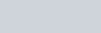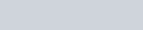##### Contact Bundaberg Park Village

Email:

```function MM_swapImgRestore(){var i,x,a=document.MM_sr;for(i=0;a&&i<a.length&&(x=a[i])&&x.oSrc;i++)x.src=x.oSrc;} function MM_preloadImages(){var d=document;if(d.images){if(!d.MM_p)d.MM_p=new Array();var i,j=d.MM_p.length,a=MM_preloadImages.arguments;for(i=0;i<a.length;i++) if(a[i].indexOf("#")!=0){d.MM_p[j]=new Image;d.MM_p[j++].src=a[i];}}} function MM_findObj(n,d){var p,i,x;if(!d)d=document;if((p=n.indexOf("?"))>0&&parent.frames.length){d=parent.frames[n.substring(p+1)].document;n=n.substring(0,p);} if(!(x=d[n])&&d.all)x=d.all[n];for(i=0;!x&&i<d.forms.length;i++)x=d.forms[i][n];for(i=0;!x&&d.layers&&i<d.layers.length;i++)x=MM_findObj(n,d.layers[i].document);if(!x&&d.getElementById)x=d.getElementById(n);return x;} function MM_swapImage(){var i,j=0,x,a=MM_swapImage.arguments;document.MM_sr=new Array;for(i=0;i<(a.length-2);i+=3) if((x=MM_findObj(a[i]))!=null){document.MM_sr[j++]=x;if(!x.oSrc)x.oSrc=x.src;x.src=a[i+2];}}```Mon – Fri: 9:00am -12:00noon
2:00pm – 5:00pm
Sat – Sun: 9:30am -2:00pm
Appointment by Arrangement